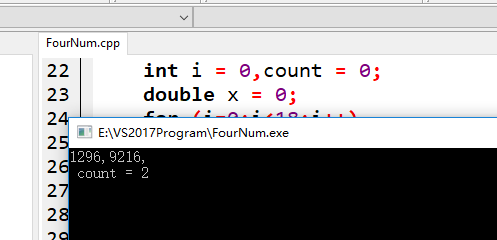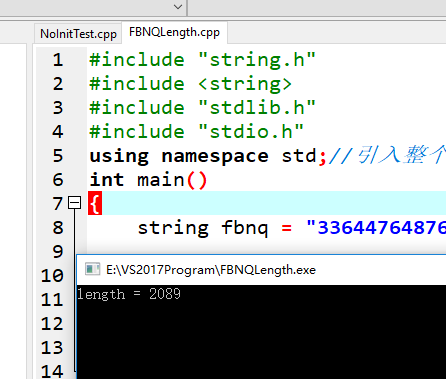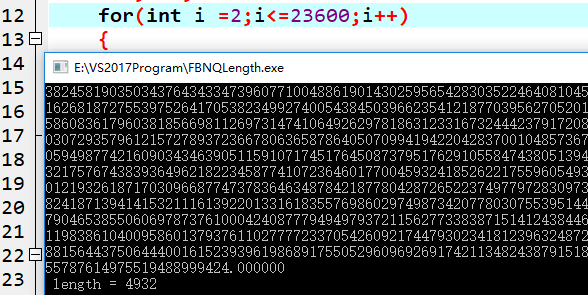2．编写程序求出满足下列条件的四位数：

(1)该数是个完全平方数；

(2)千位、十位数字之和为10且百位、个位数字之积为12。

#include
#include
int main()
{
int m,n,qw,bw,sw,gw,sum=0;
for(m=1000;m<=9999;m++)
{
for(n=32;n<=99;n++)
{
if(m==n*n)
break;
else
continue;
}
if(n<100)
{
qw=m/1000;
bw=(m%1000)/100;
sw=(m%100)/10;
gw=m%10;
if(qw+sw==10&&bw+gw==12)
{
printf("%6d",m);
sum+=1;
if(sum%5==0)
printf("\n");
}

``````}
else
continue;
``````

}
printf("\n");
printf("满足条件的数字个数有%d",sum);
return 0;
}

11个回答

`````` //2．编写程序求出满足下列条件的四位数：
//(1)该数是个完全平方数；
//(2)千位、十位数字之和为10且百位、个位数字之积为12。
//要求每一行显示5个数，并且统计满足条件的数字个数。

/*

Num = {1394,2384,3374,4364,5354,6344,7334,8324,9314,1296,2286,3276,4266,5256,6246,7236,8226,9216};

*/
//#include <stdafx.h>
#include "stdio.h"
#include "stdlib.h"
#include "math.h"
int Num = {1394,2384,3374,4364,5354,6344,7334,8324,9314,1296,2286,3276,4266,5256,6246,7236,8226,9216};
int main()
{
int i = 0,count = 0;
double x = 0;
for (i=0;i<18;i++)
{
x = sqrt(Num[i]);//开平方求根
if(x == (int)x)//判断是否是完全开尽
{
count++;
if(count % 5 == 0)
{
printf("\n");
}
printf("%d,",Num[i]);
}
}
printf("\n count = %d",count);
getchar();
return 0;
}
``````x_xx_xxx_xxxx 回复?????????(???????)??: DCBA是完全平方数，A可能的值，是由a决定。a可以是 0~9. 0*0 =0A 只能是由 a*a 得到，对吧。 0*0=0； 1*1=1; 2*2=4； 3*3=9； 4*4=16 个位数也是6 5*5=25 个位数也是5 6*6=36 个位数也是6 7*7=49 个位数也是9 8*8=64 个位数也是4 9*9=81 个位数也是1 总结下来，个位数只能是 0、1、4、5、6、9. 所以尾数是2、3的情况不存在。x_xx_xxx_xxxx 回复?????????(???????)??: 你好。我具体来解释一下。设这个数是DCBA，它是完全平方数，设它的根 为 dcba （d、c、有可能为0）。qq_37225432 我觉得不是18种可能，是36种，你把百位和各位的可能性算少了，（3,4），（4,3）,（2,6）,（6,2）

DCBA是完全平方数，A可能的值，是由a决定。a可以是 0~9. 0*0 =0A 只能是由 a*a 得到，对吧。
A 只能是由 a*a 得到，对吧。
0*0=0；
1*1=1;
2*2=4；
3*3=9；
4*4=16 个位数也是6
5*5=25 个位数也是5
6*6=36 个位数也是6
7*7=49 个位数也是9
8*8=64 个位数也是4
9*9=81 个位数也是1

10000位大概有3000位数。（n>=2，n∈N* , n∈(2,30000)）qq_37225432 然后我发现n应该是可以输入从2-30000，但是到n=45之后，就会超出无符号长整形的范围。这要怎么办哪qq_37225432 #include<stdio.h> int main(){ int a=1,b=1; long int c; int n; int i; do{printf("请输入n的值:"); scanf("%d",&n); printf("\n"); }while(n<2||n>30000); for(i=2;i<=n;i++) {c=a+b; a=b; b=c;} printf("输出第%d个斐波那契数列值为%ld\n",n,c); return 0; } 运行结果和你的要求是一致的，但是优化，没有想法。

C 中 double -1.7*10^-308~1.7*10^308
long double -1.2*10^-4932~1.2*10^4932

1.不要把思维局限在 long 、double 这些数值型输出，这仅是输出格式而已，最终体现出来都是一样。
2.你需要自己造轮子，制作小工具。

long double 也是只能支持 第 23600 位斐波那契。你再考虑考虑~